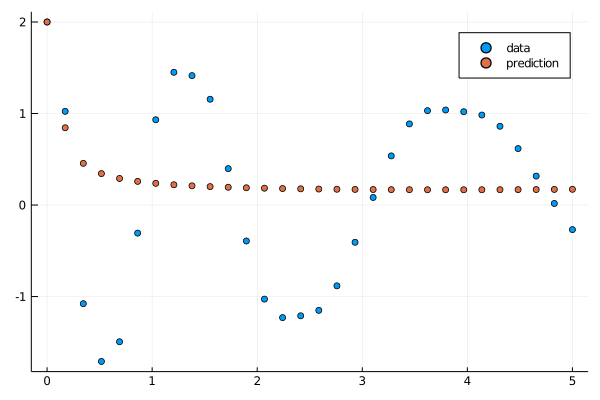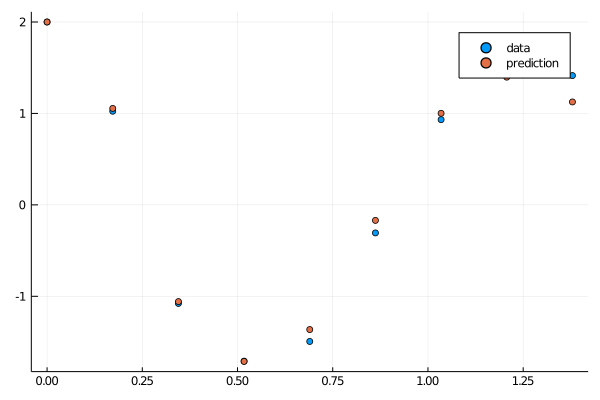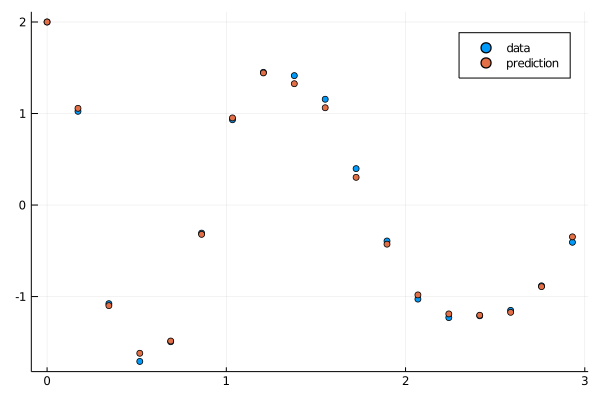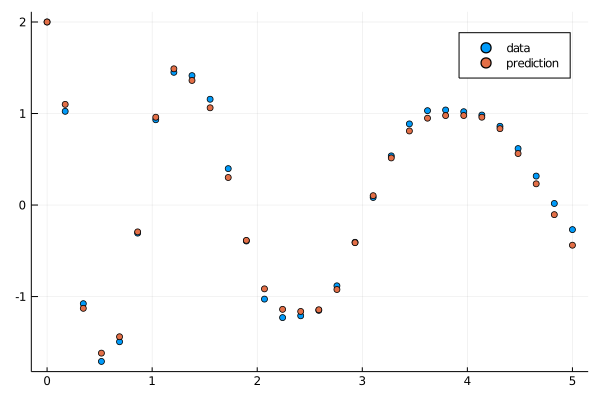# Strategies to Avoid Local Minima

Local minima can be an issue with fitting neural differential equations. However, there are many strategies to avoid local minima:

1. Insert stochasticity into the loss function through minibatching
2. Weigh the loss function to allow for fitting earlier portions first
3. Changing the optimizers to allow_f_increases
4. Iteratively grow the fit
5. Training the initial conditions and the parameters to start

## allow_f_increases=true

With Optim.jl optimizers, you can set allow_f_increases=true in order to let increases in the loss function not cause an automatic halt of the optimization process. Using a method like BFGS or NewtonTrustRegion is not guaranteed to have monotonic convergence and so this can stop early exits which can result in local minima. This looks like:

pmin = Optimization.solve(optprob, NewtonTrustRegion(), callback=callback,
maxiters = 200, allow_f_increases = true)

## Iterative Growing Of Fits to Reduce Probability of Bad Local Minima

In this example we will show how to use strategy (4) in order to increase the robustness of the fit. Let's start with the same neural ODE example we've used before except with one small twist: we wish to find the neural ODE that fits on (0,5.0). Naively, we use the same training strategy as before:

using Lux, DiffEqFlux, DifferentialEquations, Optimization, OptimizationOptimJL, Plots, Random

rng = Random.default_rng()
u0 = Float32[2.0; 0.0]
datasize = 30
tspan = (0.0f0, 5.0f0)
tsteps = range(tspan, tspan, length = datasize)

function trueODEfunc(du, u, p, t)
true_A = Float32[-0.1 2.0; -2.0 -0.1]
du .= ((u.^3)'true_A)'
end

prob_trueode = ODEProblem(trueODEfunc, u0, tspan)
ode_data = Array(solve(prob_trueode, Tsit5(), saveat = tsteps))

dudt2 = Lux.Chain(ActivationFunction(x -> x.^3),
Lux.Dense(2, 16, tanh),
Lux.Dense(16, 2))

pinit, st = Lux.setup(rng, dudt2)
pinit = Lux.ComponentArray(pinit)
prob_neuralode = NeuralODE(dudt2, tspan, Vern7(), saveat = tsteps, abstol=1e-6, reltol=1e-6)

function predict_neuralode(p)
Array(prob_neuralode(u0, p, st))
end

function loss_neuralode(p)
pred = predict_neuralode(p)
loss = sum(abs2, (ode_data[:,1:size(pred,2)] .- pred))
return loss, pred
end

iter = 0
callback = function (p, l, pred; doplot = false)
global iter
iter += 1

println(l)
if doplot
# plot current prediction against data
plt = scatter(tsteps[1:size(pred,2)], ode_data[1,1:size(pred,2)], label = "data")
scatter!(plt, tsteps[1:size(pred,2)], pred[1,:], label = "prediction")
display(plot(plt))
end

return false
end

optf = Optimization.OptimizationFunction((x,p) -> loss_neuralode(x), adtype)

optprob = Optimization.OptimizationProblem(optf, pinit)
result_neuralode = Optimization.solve(optprob,
ADAM(0.05), callback = callback,
maxiters = 300)

callback(result_neuralode.u,loss_neuralode(result_neuralode.u)...;doplot=true)However, we've now fallen into a trap of a local minima. If the optimizer changes the parameters so it dips early, it will increase the loss because there will be more error in the later parts of the time series. Thus it tends to just stay flat and never fit perfectly. This thus suggests strategies (2) and (3): do not allow the later parts of the time series to influence the fit until the later stages. Strategy (3) seems to be more robust, so this is what will be demonstrated.

Let's start by reducing the timespan to (0,1.5):

prob_neuralode = NeuralODE(dudt2, (0.0f0,1.5f0), Tsit5(), saveat = tsteps[tsteps .<= 1.5])

optf = Optimization.OptimizationFunction((x,p) -> loss_neuralode(x), adtype)

optprob = Optimization.OptimizationProblem(optf, pinit)
result_neuralode2 = Optimization.solve(optprob,
ADAM(0.05), callback = callback,
maxiters = 300)

callback(result_neuralode2.u,loss_neuralode(result_neuralode2.u)...;doplot=true)This fits beautifully. Now let's grow the timespan and utilize the parameters from our (0,1.5) fit as the initial condition to our next fit:

prob_neuralode = NeuralODE(dudt2, (0.0f0,3.0f0), Tsit5(), saveat = tsteps[tsteps .<= 3.0])

optprob = Optimization.OptimizationProblem(optf, result_neuralode.u)
result_neuralode3 = Optimization.solve(optprob,
ADAM(0.05), maxiters = 300,
callback = callback)
callback(result_neuralode3.u,loss_neuralode(result_neuralode3.u)...;doplot=true)Once again a great fit. Now we utilize these parameters as the initial condition to the full fit:

prob_neuralode = NeuralODE(dudt2, (0.0f0,5.0f0), Tsit5(), saveat = tsteps)
optprob = Optimization.OptimizationProblem(optf, result_neuralode3.u)
result_neuralode4 = Optimization.solve(optprob,
ADAM(0.01), maxiters = 300,
callback = callback)
callback(result_neuralode4.u,loss_neuralode(result_neuralode4.u)...;doplot=true)## Training both the initial conditions and the parameters to start

In this example we will show how to use strategy (5) in order to accomplish the same goal, except rather than growing the trajectory iteratively, we can train on the whole trajectory. We do this by allowing the neural ODE to learn both the initial conditions and parameters to start, and then reset the initial conditions back and train only the parameters. Note: this strategy is demonstrated for the (0, 5) time span and (0, 10), any longer and more iterations will be required. Alternatively, one could use a mix of (4) and (5), or breaking up the trajectory into chunks and just (5).


using Flux, Plots, DifferentialEquations, SciMLSensitivity

#Starting example with tspan (0, 5)
u0 = Float32[2.0; 0.0]
datasize = 30
tspan = (0.0f0, 5.0f0)
tsteps = range(tspan, tspan, length = datasize)

function trueODEfunc(du, u, p, t)
true_A = [-0.1 2.0; -2.0 -0.1]
du .= ((u.^3)'true_A)'
end

prob_trueode = ODEProblem(trueODEfunc, u0, tspan)
ode_data = Array(solve(prob_trueode, Tsit5(), saveat = tsteps))

#Using flux here to easily demonstrate the idea, but this can be done with Optimization.solve!
dudt2 = Chain(Dense(2,16, tanh),
Dense(16,2))

p,re = Flux.destructure(dudt2) # use this p as the initial condition!
dudt(u,p,t) = re(p)(u) # need to restrcture for backprop!
prob = ODEProblem(dudt,u0,tspan)

function predict_n_ode()
Array(solve(prob,u0=u0,p=p, saveat=tsteps))
end

function loss_n_ode()
pred = predict_n_ode()
sqnorm(x) = sum(abs2, x)
loss = sum(abs2,ode_data .- pred)
loss
end

function callback(;doplot=true) #callback function to observe training
pred = predict_n_ode()
display(sum(abs2,ode_data .- pred))
if doplot
# plot current prediction against data
pl = plot(tsteps,ode_data[1,:],label="data")
plot!(pl,tsteps,pred[1,:],label="prediction")
display(plot(pl))
end
return false
end
predict_n_ode()
loss_n_ode()
callback()

data = Iterators.repeated((), 1000)

#Specify to flux to include both the initial conditions (IC) and parameters of the NODE to train
Flux.train!(loss_n_ode, Flux.params(u0, p), data,
Flux.Optimise.ADAM(0.05), cb = callback)

#Here we reset the IC back to the original and train only the NODE parameters
u0 = Float32[2.0; 0.0]
Flux.train!(loss_n_ode, Flux.params(p), data,
Flux.Optimise.ADAM(0.05), cb = callback)

callback()

#Now use the same technique for a longer tspan (0, 10)
datasize = 30
tspan = (0.0f0, 10.0f0)
tsteps = range(tspan, tspan, length = datasize)

prob_trueode = ODEProblem(trueODEfunc, u0, tspan)
ode_data = Array(solve(prob_trueode, Tsit5(), saveat = tsteps))

dudt2 = Chain(Dense(2,16, tanh),
Dense(16,2))

p,re = Flux.destructure(dudt2) # use this p as the initial condition!
dudt(u,p,t) = re(p)(u) # need to restrcture for backprop!
prob = ODEProblem(dudt,u0,tspan)

data = Iterators.repeated((), 1500)

Flux.train!(loss_n_ode, Flux.params(u0, p), data,
Flux.Optimise.ADAM(0.05), cb = callback)

u0 = Float32[2.0; 0.0]
Flux.train!(loss_n_ode, Flux.params(p), data,
Flux.Optimise.ADAM(0.05), cb = callback)

callback()


And there we go, a set of robust strategies for fitting an equation that would otherwise get stuck in a local optima.